Test: Self Inductance - 2

# Test: Self Inductance - 2

Test Description

## 10 Questions MCQ Test Electromagnetic Fields Theory (EMFT) | Test: Self Inductance - 2

Test: Self Inductance - 2 for Electrical Engineering (EE) 2023 is part of Electromagnetic Fields Theory (EMFT) preparation. The Test: Self Inductance - 2 questions and answers have been prepared according to the Electrical Engineering (EE) exam syllabus.The Test: Self Inductance - 2 MCQs are made for Electrical Engineering (EE) 2023 Exam. Find important definitions, questions, notes, meanings, examples, exercises, MCQs and online tests for Test: Self Inductance - 2 below.
Solutions of Test: Self Inductance - 2 questions in English are available as part of our Electromagnetic Fields Theory (EMFT) for Electrical Engineering (EE) & Test: Self Inductance - 2 solutions in Hindi for Electromagnetic Fields Theory (EMFT) course. Download more important topics, notes, lectures and mock test series for Electrical Engineering (EE) Exam by signing up for free. Attempt Test: Self Inductance - 2 | 10 questions in 45 minutes | Mock test for Electrical Engineering (EE) preparation | Free important questions MCQ to study Electromagnetic Fields Theory (EMFT) for Electrical Engineering (EE) Exam | Download free PDF with solutions
 1 Crore+ students have signed up on EduRev. Have you?
Test: Self Inductance - 2 - Question 1

### In a coil of N turns, a current of i produces a magnetic flux of ϕ. Find the self inductance of this coil.

Detailed Solution for Test: Self Inductance - 2 - Question 1

Concept:

• Self-inductance: The property of the current-carrying coil that opposes or resists the change of current flowing through itself is called self-inductance.

It is calculated by: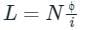where N is the number of turns, ϕ is the magnetic flux, i is current, and L is the self-inductance.

Calculation:
The self-inductance of a coil is calculated by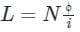So the correct answer is option 3.

Test: Self Inductance - 2 - Question 2

### Two inductors when connected in series with additive connection, their equivalent inductance is 400 µH and when same inductors connected in series with subtractive connection, their equivalent inductance is 300 µH. Find their mutual inductance.

Detailed Solution for Test: Self Inductance - 2 - Question 2

Let two inductors are L1 and L2 and their mutual inductance is M.
When both inductors are in additive connection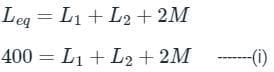When both inductors are in subtractive connection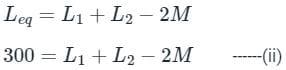Subtracting equation (i) and (ii)
100 = 4M
M = 100/4 = 25 µH
Mutual inductance will 25 µH.

Test: Self Inductance - 2 - Question 3

### If N is the number of turns in a coil, the value of self inductance varies as

Detailed Solution for Test: Self Inductance - 2 - Question 3

Concept:

Self-Induction

• Whenever the electric current passing through a coil changes, the magnetic flux linked with it will also change.
• As a result of this, in accordance with Faraday’s laws of electromagnetic induction, an emf is induced in the coil which opposes the change that causes it.
• This phenomenon is called ‘self-induction’ and the emf induced is called back emf, current so produced in the coil is called induced current.
• Self-inductance of a solenoid is given by –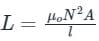Where μo = Absolute permeability, N = Number of turns, l = length of the solenoid and A = Area of the solenoid.

Explanation:

We know self-inductance,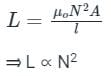Hence the correct answer is N2

Test: Self Inductance - 2 - Question 4

A current in a coil of inductance 5 H decreases at a rate of 2 Amp/sec. The induced emf is

Detailed Solution for Test: Self Inductance - 2 - Question 4

Concept:
Self-Induction:

• Whenever the electric current passing through a coil changes, the magnetic flux linked with it will also change.
• As a result of this, in accordance with Faraday’s laws of electromagnetic induction, an emf is induced in the coil which opposes the change that causes it.
• This phenomenon is called ‘self-induction’ and the emf induced is called back emf, current so produced in the coil is called induced current.
• Self-inductance of a solenoid is given by –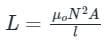Where μo = Absolute permeability, N = Number of turns, l = length of the solenoid, the resistance of the coil (R) = 4Ω, and A = Area of the solenoid.
Induced e.m.f can be given as,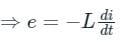Calculation
Given -
di/dt = -2 Amp/sec and L = 5 H (Since the current decreases at a rate of 2 Amp/sec).
The emf induced across the inductor is,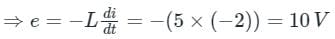Test: Self Inductance - 2 - Question 5

Current in a coil changes from 4 A to zero in 0.1 second and the emf induced is 100 V. The self inductance of the coil is

Detailed Solution for Test: Self Inductance - 2 - Question 5

The correct answer is option 2) i.e. 2.5 H.

Concept:

• Self-inductance: Self-inductance is the phenomenon where a current-carrying coil resists the change in the current flowing through it.
• ​The resistance to the change in current is due to the induced emf in the coil.
• The direction of the induced emf is opposite to the applied voltage if the current is increasing and the direction of the induced emf is in the same direction as the applied voltage if the current is decreasing.
• The unit of inductance is Henry (H).

The induced emf is related to the change in current as follows: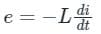Where e is the induced emf, L is the self-inductance of the coil, and di/dt is the rate of change of current in the coil.

Calculation:
Given that:
Change in current, di = (0 - 4) A
Time interval, dt = 0.1 s
Induced emf, e = 100 V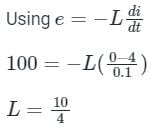Self-inductance, L = 2.5 H​​​​​

Test: Self Inductance - 2 - Question 6

In a coil, the current changes from 4A to 2A in 0.05 sec. If the induced e.m.f. is 8 volt, then self-inductance of the coil is:

Detailed Solution for Test: Self Inductance - 2 - Question 6

Concept:

Self-Induction

• Whenever the electric current passing through a coil changes, the magnetic flux linked with it will also change.
• As a result of this, in accordance with Faraday’s laws of electromagnetic induction, an emf is induced in the coil which opposes the change that causes it.
• This phenomenon is called ‘self-induction’ and the emf induced is called back emf, current so produced in the coil is called induced current.
• Self-inductance of a solenoid is given by –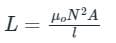Where μo = Absolute permeability, N = Number of turns, l = length of the solenoid, the resistance of the coil (R) = 4Ω, and A = Area of the solenoid.

Induced e.m.f can be given as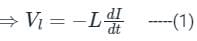Where,
VL = induced voltage in volts
N = self-inductance of the coil
dI/dt= rate of change of current in ampere/second

Calculation:
Given I1 = 4A, I2 = 2A, dt = 0.05 sec, VL = 8 volt
⇒ dI = I2 - I1 = (2 - 4) = -2A

From equation 1,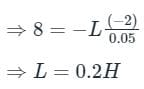Hence, option 3 is correct.

Test: Self Inductance - 2 - Question 7

The self inductance of a long solenoid of length l is proportional to

Detailed Solution for Test: Self Inductance - 2 - Question 7

Explanation:
Solenoid:

• The solenoid is a long cylindrical coil of wire consisting of a large number of turns bound together very tightly.

Self-Induction:

• Whenever the electric current passing through a coil changes, the magnetic flux linked with it will also change.
• As a result of this, in accordance with Faraday’s laws of electromagnetic induction, an emf is induced in the coil which opposes the change that causes it.
• This phenomenon is called ‘self-induction’ and the emf induced is called back emf, current so produced in the coil is called induced current.

Self-inductance of a solenoid is given by: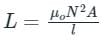where μo = Absolute permeability, N = Number of turns, l = length of the solenoid, and A = cross-sectional area of the solenoid.

Thus we can say that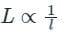Test: Self Inductance - 2 - Question 8

When the current changes from +2A to -2A in 0.05 second, an emf of 8V is induced in the coil. The coefficient of self inductance of the coil is

Detailed Solution for Test: Self Inductance - 2 - Question 8

Concept:

Self-Induction

• Whenever the electric current passing through a coil changes, the magnetic flux linked with it will also change.
• As a result of this, in accordance with Faraday’s laws of electromagnetic induction, an emf is induced in the coil which opposes the change that causes it.
• This phenomenon is called ‘self-induction’ and the emf induced is called back emf, current so produced in the coil is called induced current.

Induced e.m.f can be given as: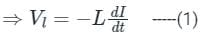Where VL = induced voltage in volts, L = self-inductance of the coil, dI/dt = rate of change of current in ampere/second.

Calculation:
Given:
I1 = +2 Amp, I2 = -2 Amp, dt = 0.05 sec, VL = 8 volt
⇒ dI = I2 - I1 = (-2 - 2) = -4 Amp
From equation 1,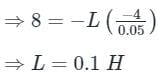Test: Self Inductance - 2 - Question 9

A circuit possesses an inductance of 1 H when a current through coil is changing uniformly at the rate of 1 A/s inducing an opposing emf of 'X' volts in it.

What is the value of 'X'?

Detailed Solution for Test: Self Inductance - 2 - Question 9

Concept:

Self-Induction

• Whenever the electric current passing through a coil changes, the magnetic flux linked with it will also change.
• As a result of this, in accordance with Faraday’s laws of electromagnetic induction, an emf is induced in the coil which opposes the change that causes it.
• This phenomenon is called ‘self-induction’ and the emf induced is called back emf, current so produced in the coil is called induced current.
• Self-inductance of a solenoid is given by –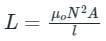Where μo = Absolute permeability, N = Number of turns, l = length of the solenoid, and A = Area of the solenoid.

Induced e.m.f can be given as
Calculation:
Given:
L = 1 H, di / dt = 1 A/s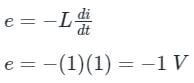Here negative sigh indicated the nature of opposing.
∴ The opposing emf X = 1 V

Test: Self Inductance - 2 - Question 10

In a coil current changes from 2A to 4A in 0.05 second. If the average induced e.m.f. is 8 volt, then coefficient of self inductance is:

Detailed Solution for Test: Self Inductance - 2 - Question 10

Concept:

Self-Induction

• Whenever the electric current passing through a coil changes, the magnetic flux linked with it will also change.
• As a result of this, in accordance with Faraday’s laws of electromagnetic induction, an emf is induced in the coil which opposes the change that causes it.
• This phenomenon is called ‘self-induction’ and the emf induced is called back emf, current so produced in the coil is called induced current.
• Self-inductance of a solenoid is given by –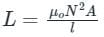Where μo = Absolute permeability, N = Number of turns, l = length of the solenoid, the resistance of the coil (R) = 4Ω, and A = Area of the solenoid.
• Induced e.m.f can be given as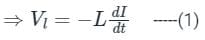Where ,
VL = induced voltage in volts
N = self-inductance of the coil
dI/dt = rate of change of current in ampere/second

Calculation:
Given I1 = 4A, I2 = 2A, dt = 0.05 sec, VL = 8 volt
⇒ dI = I2 - I1 = (2 - 4) = -2A
From equation 1,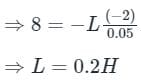Hence, option 1 is correct.

## Electromagnetic Fields Theory (EMFT)

11 videos|46 docs|62 tests
 Use Code STAYHOME200 and get INR 200 additional OFF Use Coupon Code
Information about Test: Self Inductance - 2 Page
In this test you can find the Exam questions for Test: Self Inductance - 2 solved & explained in the simplest way possible. Besides giving Questions and answers for Test: Self Inductance - 2, EduRev gives you an ample number of Online tests for practice

## Electromagnetic Fields Theory (EMFT)

11 videos|46 docs|62 tests